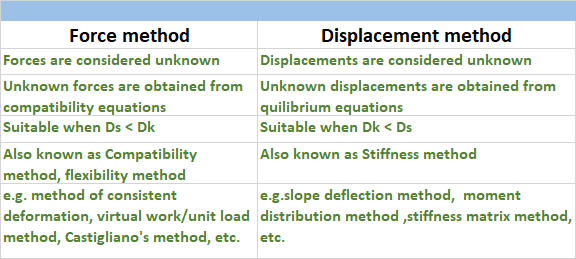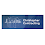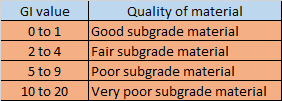## Translate

### Difference between Force method and Displacement method of analysis of structure

•  For an indeterminate structure,  the condition of static equilibrium equations are not sufficient to analyze the structure.  To analyze an indeterminate structure,  some additional equilibrium or compatibility equations are required.
• In force method of analysis of indeterminate structure,  the unknowns are taken as forces (i.e, member forces or reaction). While in case of displacement method of analysis of indeterminate structure, the unknowns are taken as displacement (i.e, Δ or ө).
• In force method of analysis of indeterminate structure,  first force displacement equations are written and then unknown forces are calculated from compatibility criteria. While in case of displacement method of analysis of indeterminate structure, first force displacement equations are written and then unknown displacement are calculated from equilibrium equations at joints.
• Force method of analysis of indeterminate structure is suitable when degree of redundancy is less, i.e, degree of static Indeterminacy is less than degree of kinematic Indeterminacy.  While displacement method of analysis of indeterminate structure is suitable when degree of freedom is less, i.e, degree of kinematic Indeterminacy is less than degree of static Indeterminacy.Difference between Force method and Displacement method of analysis

1.I liked your work and, as a result, the manner you presented this content about christopher foundation.It is a valuable paper for us. Thank you for sharing this blog with us.

2.This comment has been removed by a blog administrator.

### Group index of soil

Group index of soil is a number used to refer the quality of soil used as a subgrade material in highway construction.  Group index of soil ...